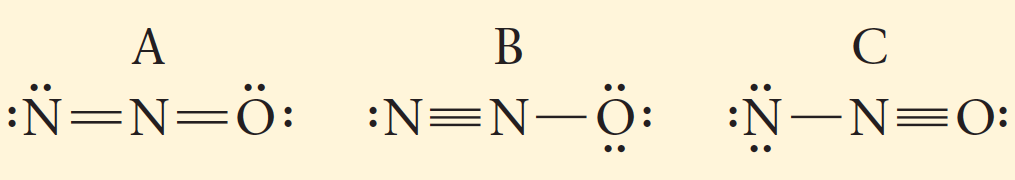# Problem: Which resonance form is likely to contribute most to the correct structure of N2O?

###### FREE Expert Solution

A

N: F.C. = 5 – (2+4) = -1

N: F.C. = 5 – (4+0) = +1

O: F.C. = 6 – (2+4) = 0

Total F.C. = 0

B

N: F.C. = 5 – (3+2) = 0

N: F.C. = 5 – (4+0) = +1

O: F.C. = 6 – (1+6) = -1

Total F.C. =  0

81% (87 ratings)###### Problem Details

Which resonance form is likely to contribute most to the correct structure of N2O?Frequently Asked Questions

What scientific concept do you need to know in order to solve this problem?

Our tutors have indicated that to solve this problem you will need to apply the Resonance Structures concept. You can view video lessons to learn Resonance Structures. Or if you need more Resonance Structures practice, you can also practice Resonance Structures practice problems.

What professor is this problem relevant for?

Based on our data, we think this problem is relevant for Professor DesEnfants' class at MONTANA.